• Opencv重载了运算符“*”，姑且称之为Mat矩阵“点乘”，其中一个重载声明为： CV_EXPORTS MatExpr operator * (const Mat& a, const Mat& b); 点乘说明： 1. A*B是以数学运算矩阵相乘的方式实现的，即Mat...

Mat矩阵点乘——A*B

Opencv重载了运算符“*”，姑且称之为Mat矩阵“点乘”，其中一个重载声明为：

CV_EXPORTS MatExpr operator * (const Mat& a, const Mat& b);

点乘说明：

1.  A*B是以数学运算中矩阵相乘的方式实现的，即Mat矩阵A和B被当做纯粹的矩阵做乘法运算，这就要求A的列数等       于B的行数时，才能定义两个矩阵相乘。如A是m×n矩阵，B是n×p矩阵，它们的乘积AB是一个m×p矩阵。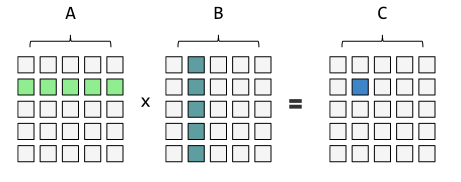如上图所示，C=AB。C中第i行第j列所在元素C(i,j)等于A中第i行所有元素跟B中第j列所有元素一一对应的乘积之和。
更具有代表性的的：对于A、B都是2行2列矩阵的情况：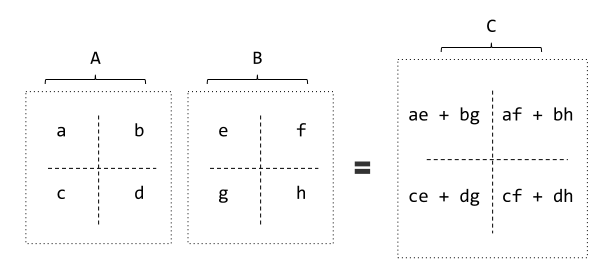Opencv验证：
定义两个Mat矩阵A和B点乘，A为2行3列，B为3行2列：

#include "core/core.hpp"
#include "iostream"

using namespace std;
using namespace cv;

int main(int argc,char *argv[])
{
Mat A=Mat::ones(2,3,CV_32FC1);
Mat B=Mat::ones(3,2,CV_32FC1);
Mat AB;

A.at<float>(0,0)=1;
A.at<float>(0,1)=2;
A.at<float>(0,2)=3;
A.at<float>(1,0)=4;
A.at<float>(1,1)=5;
A.at<float>(1,2)=6;

B.at<float>(0,0)=1;
B.at<float>(0,1)=2;
B.at<float>(1,0)=3;
B.at<float>(1,1)=4;
B.at<float>(2,0)=5;
B.at<float>(2,1)=6;

AB=A*B;

cout<<"A=\n"<<A<<endl<<endl;
cout<<"B=\n"<<B<<endl<<endl;
cout<<"AB=\n"<<AB<<endl<<endl;

system("pause");
}

输出：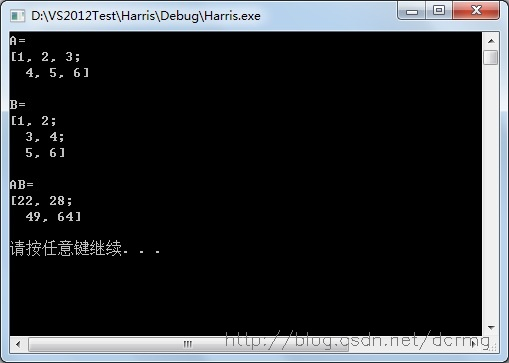务必保证两个Mat矩阵中第一个矩阵A的列数等于第二个矩阵B的行数。

2.  参与点乘的两个Mat矩阵的数据类型（type）只能是 CV_32F、 CV_64FC1、 CV_32FC2、 CV_64FC2 这4种类        型中的一种。若选用其他类型，比如CV_8UC1，编译器会报错：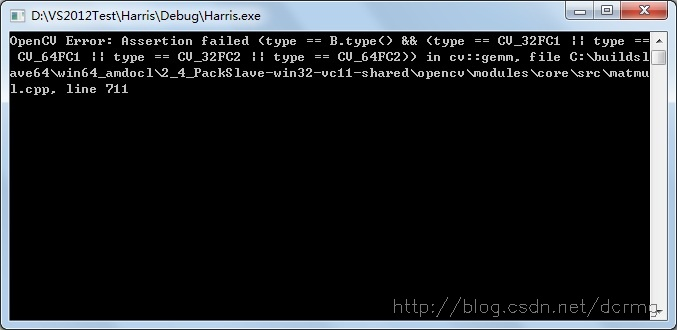Mat矩阵dot——A.dot(B)

Opencv中.dot操作才算得上是真正的“点乘”，A.dot(B)操作相当于数学向量运算中的点乘，也叫向量的内积、数量积。

函数声明：

  //! computes dot-product
double dot(InputArray m) const;

dot说明：

1.  对两个向量执行点乘运算，就是对这两个向量对应位一一相乘之后求和的操作，点乘的结果是一个标量。

对于向量a和向量b：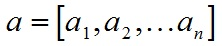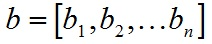a和b的点积公式为：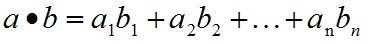要求向量a和向量b的行列数相同。

Mat矩阵的dot方法扩展了一维向量的点乘操作，把整个Mat矩阵扩展成一个行（列）向量，之后执行向量的点乘运算，仍然要求参与dot运算的两个Mat矩阵的行列数完全一致。

2.  dot方法声明中显示返回值是double，所以A.dot(B)结果是一个double类型数据，不是Mat矩阵，不能把A.dot(B)结       果赋值给Mat矩阵！

Opencv验证：

#include "core/core.hpp"
#include "iostream"

using namespace std;
using namespace cv;

int main(int argc,char *argv[])
{
Mat A=Mat::ones(2,3,CV_8UC1);
Mat B=Mat::ones(2,3,CV_8UC1);

A.at<uchar>(0,0)=1;
A.at<uchar>(0,1)=2;
A.at<uchar>(0,2)=3;
A.at<uchar>(1,0)=4;
A.at<uchar>(1,1)=5;
A.at<uchar>(1,2)=6;

B.at<uchar>(0,0)=1;
B.at<uchar>(0,1)=2;
B.at<uchar>(0,2)=3;
B.at<uchar>(1,0)=4;
B.at<uchar>(1,1)=5;
B.at<uchar>(1,2)=6;

double AB=A.dot(B);

cout<<"A=\n"<<A<<endl<<endl;
cout<<"B=\n"<<B<<endl<<endl;
cout<<"double类型的AB=\n"<<AB<<endl<<endl;

system("pause");
}


运行结果：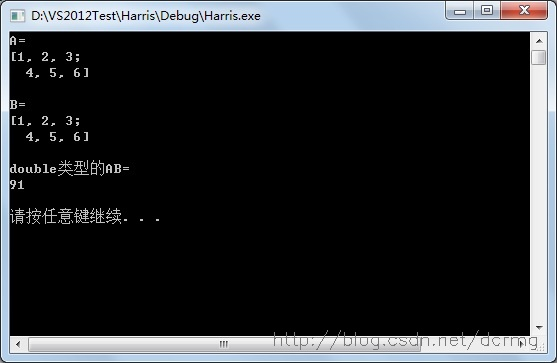若对AB声明为Mat，则在编译阶段就会报错。

3.  dot操作不对参与运算的矩阵A、B的数据类型做要求，CV_8UC1、CV_32FC1等，可以是任何Opencv定义的类         型，如在2中使用的就是CV_8UC1。

4.  若参与dot运算的两个Mat矩阵是多通道的，则计算结果是所有通道单独计算各自.dot之后，再累计的和，结果仍是一个double类型数据。

Mat矩阵mul——A.mul(B)

Opencv中mul会计算两个Mat矩阵对应位的乘积，所以要求参与运算的矩阵A的行列和B的行列数一致。计算结果是跟A或B行列数一致的一个Mat矩阵。

Opencv中mul声明：

//! per-element matrix multiplication by means of matrix expressions
MatExpr mul(InputArray m, double scale=1) const;

以简单的情况为例，对于2*2大小的Mat矩阵A和B：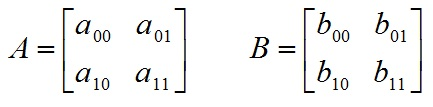对A和B执行mul运算：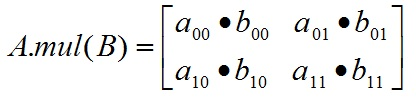mul说明：

1.  mul操作不对参与运算的两个矩阵A、B有数据类型上的要求，但要求A，B类型一致，不然报错；
2.  Mat AB=A.mul(B)，若声明AB时没有定义AB的数据类型，则默认AB的数据类型跟A和B保存一致；
3.  若AB精度不够，可能产生溢出，溢出的值被置为当前精度下的最大值；

Opencv验证：

#include "core/core.hpp"
#include "iostream"

using namespace std;
using namespace cv;

int main(int argc,char *argv[])
{
Mat A=Mat::ones(2,3,CV_8UC1);
Mat B=Mat::ones(2,3,CV_8UC1);

A.at<uchar>(0,0)=60;
A.at<uchar>(0,1)=2;
A.at<uchar>(0,2)=3;
A.at<uchar>(1,0)=4;
A.at<uchar>(1,1)=5;
A.at<uchar>(1,2)=6;

B.at<uchar>(0,0)=60;
B.at<uchar>(0,1)=2;
B.at<uchar>(0,2)=3;
B.at<uchar>(1,0)=4;
B.at<uchar>(1,1)=5;
B.at<uchar>(1,2)=6;

Mat AB=A.mul(B);

cout<<"A=\n"<<A<<endl<<endl;
cout<<"B=\n"<<B<<endl<<endl;
cout<<"AB=\n"<<AB<<endl<<endl;

system("pause");
}


输出：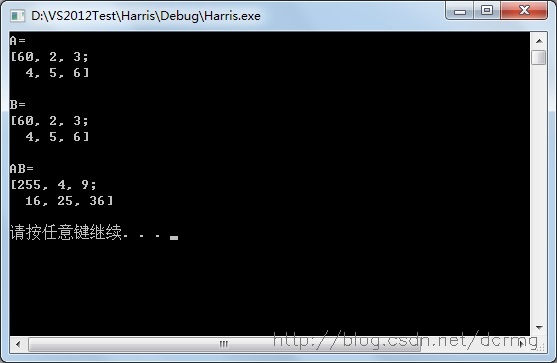AB中第一个元素应该为60*60=360，但AB默认的类型为CV_8UC1,即最大值只能是255；所以执行mul运算一定要定义AB足够的精度，防止溢出。

展开全文矩阵相乘 点乘
• Opencv重载了运算符“*”，称之为Mat矩阵乘法，其中一个重载声明为： CV_EXPORTS MatExpr operator * (const Mat& a, const Mat& b); 点乘说明： A*B是以数学运算矩阵相乘的方式实现的，即Mat矩阵A和B被...
Mat矩阵乘积——A*B
Opencv重载了运算符“*”，称之为Mat矩阵乘法，其中一个重载声明为：
CV_EXPORTS MatExpr operator * (const Mat& a, const Mat& b);

点乘说明：
A*B是以数学运算中矩阵相乘的方式实现的，即Mat矩阵A和B被当做纯粹的矩阵做乘法运算，这就要求A的列数等 于B的行数时，才能定义两个矩阵相乘。如A是m×n矩阵，B是n×p矩阵，它们的乘积AB是一个m×p矩阵。参与点乘的两个Mat矩阵的数据类型（type）只能是 CV_32F、 CV_64FC1、 CV_32FC2、 CV_64FC2 这4种类 型中的一种。若选用其他类型，比如CV_8UC1，编译器会报错：
Opencv验证：
定义两个Mat矩阵A和B点乘，A为2行3列，B为3行2列：
#include "core/core.hpp"
#include "iostream"

using namespace std;
using namespace cv;

int main(int argc,char *argv[])
{
Mat A=Mat::ones(2,3,CV_32FC1);
Mat B=Mat::ones(3,2,CV_32FC1);
Mat AB;

A.at<float>(0,0)=1;
A.at<float>(0,1)=2;
A.at<float>(0,2)=3;
A.at<float>(1,0)=4;
A.at<float>(1,1)=5;
A.at<float>(1,2)=6;

B.at<float>(0,0)=1;
B.at<float>(0,1)=2;
B.at<float>(1,0)=3;
B.at<float>(1,1)=4;
B.at<float>(2,0)=5;
B.at<float>(2,1)=6;

AB=A*B;

cout<<"A=\n"<<A<<endl<<endl;
cout<<"B=\n"<<B<<endl<<endl;
cout<<"AB=\n"<<AB<<endl<<endl;

system("pause");
}

务必保证两个Mat矩阵中第一个矩阵A的列数等于第二个矩阵B的行数。
Mat矩阵dot——A.dot(B)
Opencv中.dot操作才算得上是真正的“点乘”，A.dot(B)操作相当于数学向量运算中的点乘，也叫向量的内积、数量积。
函数声明：
//! computes dot-product
double dot(InputArray m) const;

dot说明：
对两个Mat类矩阵执行点乘运算，就是把整个Mat矩阵扩展成一个行（列）向量，之后执行向量的点乘运算，对应位一一相乘之后求和的操作，点乘的结果是一个标量。  dot方法声明中显示返回值是double，所以A.dot(B)结果是一个double类型数据，不是Mat矩阵，不能把A.dot(B)结 果赋值给Mat矩阵！  dot操作不对参与运算的矩阵A、B的数据类型做要求，CV_8UC1、CV_32FC1等，可以是任何Opencv定义的类 型，如在2中使用的就是CV_8UC1。  若参与dot运算的两个Mat矩阵是多通道的，则计算结果是所有通道单独计算各自.dot之后，再累计的和，结果仍是一个double类型数据。
Opencv验证：
#include "core/core.hpp"
#include "iostream"

using namespace std;
using namespace cv;

int main(int argc,char *argv[])
{
Mat A=Mat::ones(2,3,CV_8UC1);
Mat B=Mat::ones(2,3,CV_8UC1);

A.at<uchar>(0,0)=1;
A.at<uchar>(0,1)=2;
A.at<uchar>(0,2)=3;
A.at<uchar>(1,0)=4;
A.at<uchar>(1,1)=5;
A.at<uchar>(1,2)=6;

B.at<uchar>(0,0)=1;
B.at<uchar>(0,1)=2;
B.at<uchar>(0,2)=3;
B.at<uchar>(1,0)=4;
B.at<uchar>(1,1)=5;
B.at<uchar>(1,2)=6;

double AB=A.dot(B);

cout<<"A=\n"<<A<<endl<<endl;
cout<<"B=\n"<<B<<endl<<endl;
cout<<"double类型的AB=\n"<<AB<<endl<<endl;

system("pause");
}

Mat矩阵mul——A.mul(B)
Opencv中mul会计算两个Mat矩阵对应位的乘积，所以要求参与运算的矩阵A的行列和B的行列数一致。计算结果是跟A或B行列数一致的一个Mat矩阵。
Opencv中mul声明：
//! per-element matrix multiplication by means of matrix expressions
MatExpr mul(InputArray m, double scale=1) const;

mul说明：
mul操作不对参与运算的两个矩阵A、B有数据类型上的要求，但要求A，B类型一致，不然报错；  Mat AB=A.mul(B)，若声明AB时没有定义AB的数据类型，则默认AB的数据类型跟A和B保存一致；  若AB精度不够，可能产生溢出，溢出的值被置为当前精度下的最大值；
Opencv验证：
#include "core/core.hpp"
#include "iostream"

using namespace std;
using namespace cv;

int main(int argc,char *argv[])
{
Mat A=Mat::ones(2,3,CV_8UC1);
Mat B=Mat::ones(2,3,CV_8UC1);

A.at<uchar>(0,0)=60;
A.at<uchar>(0,1)=2;
A.at<uchar>(0,2)=3;
A.at<uchar>(1,0)=4;
A.at<uchar>(1,1)=5;
A.at<uchar>(1,2)=6;

B.at<uchar>(0,0)=60;
B.at<uchar>(0,1)=2;
B.at<uchar>(0,2)=3;
B.at<uchar>(1,0)=4;
B.at<uchar>(1,1)=5;
B.at<uchar>(1,2)=6;

Mat AB=A.mul(B);

cout<<"A=\n"<<A<<endl<<endl;
cout<<"B=\n"<<B<<endl<<endl;
cout<<"AB=\n"<<AB<<endl<<endl;

system("pause");
}

输出：
AB中第一个元素应该为60*60=360，但AB默认的类型为CV_8UC1,即最大值只能是255；所以执行mul运算一定要定义AB足够的精度，防止溢出
展开全文计算机视觉
• Opencv重载了运算符“*”，姑且称之为Mat矩阵“点乘”，其中一个重载声明为： [cpp] view plain copy  print? CV_EXPORTS MatExpr operator * (const Mat& a, const Mat& b); ...
1、Mat矩阵点乘——A*B

Opencv重载了运算符“*”，姑且称之为Mat矩阵“点乘”，其中一个重载声明为：

[cpp]
view plain
copy

print
?

CV_EXPORTS MatExpr operator * (const Mat& a, const Mat& b);

点乘说明：

1.  A*B是以数学运算中矩阵相乘的方式实现的，即Mat矩阵A和B被当做纯粹的矩阵做乘法运算，这就要求A的列数等       于B的行数时，才能定义两个矩阵相乘。如A是m×n矩阵，B是n×p矩阵，它们的乘积AB是一个m×p矩阵。如上图所示，C=AB。C中第i行第j列所在元素C(i,j)等于A中第i行所有元素跟B中第j列所有元素一一对应的乘积之和。
更具有代表性的的：对于A、B都是2行2列矩阵的情况：

务必保证两个Mat矩阵中第一个矩阵A的列数等于第二个矩阵B的行数。参与点乘的两个Mat矩阵的数据类型（type）只能是 CV_32F、 CV_64FC1、 CV_32FC2、 CV_64FC2 这4种类        型中的一种。若选用其他类型，比如CV_8UC1，编译器会报错。
2、Mat矩阵dot——A.dot(B)
1）Opencv中.dot操作才算得上是真正的“点乘”，A.dot(B)操作相当于数学向量运算中的点乘，也叫向量的内积、数量积。

函数声明：

[cpp]
view plain
copy

print
?

//! computes dot-product     double dot(InputArray m) const;

dot说明：

1.  对两个向量执行点乘运算，就是对这两个向量对应位一一相乘之后求和的操作，点乘的结果是一个标量。

对于向量a和向量b：a和b的点积公式为：要求向量a和向量b的行列数相同。

Mat矩阵的dot方法扩展了一维向量的点乘操作，把整个Mat矩阵扩展成一个行（列）向量，之后执行向量的点乘运算，仍然要求参与dot运算的两个Mat矩阵的行列数完全一致。
2）dot方法声明中显示返回值是double，所以A.dot(B)结果是一个double类型数据，不是Mat矩阵，不能把A.dot(B)结       果赋值给Mat矩阵！
3）dot操作不对参与运算的矩阵A、B的数据类型做要求，CV_8UC1、CV_32FC1等，可以是任何Opencv定义的类         型，如在2中使用的就是CV_8UC1。
4）若参与dot运算的两个Mat矩阵是多通道的，则计算结果是所有通道单独计算各自.dot之后，再累计的和，结果仍是一个double类型数据。
3、Mat矩阵mul——A.mul(B)

Opencv中mul会计算两个Mat矩阵对应位的乘积，所以要求参与运算的矩阵A的行列和B的行列数一致。计算结果是跟A或B行列数一致的一个Mat矩阵。

Opencv中mul声明：

[cpp]
view plain
copy

print
?

//! per-element matrix multiplication by means of matrix expressions       MatExpr mul(InputArray m, double scale=1) const;

以简单的情况为例，对于2*2大小的Mat矩阵A和B：对A和B执行mul运算：mul说明：

1）mul操作不对参与运算的两个矩阵A、B有数据类型上的要求，但要求A，B类型一致，不然报错；
2） Mat AB=A.mul(B)，若声明AB时没有定义AB的数据类型，则默认AB的数据类型跟A和B保存一致；
3）若AB精度不够，可能产生溢出，溢出的值被置为当前精度下的最大值； 转载自：http://blog.csdn.net/dcrmg/article/details/52404580

展开全文算法 应用
• 原博客：... Mat矩阵点乘——A*B ...Opencv重载了运算符“*”，姑且称之为Mat矩阵“点乘”，其中一个重载声明为： CV_EXPORTS MatExpr operator * (const Mat& a, const Mat& b);
原博客：http://blog.csdn.net/dcrmg/article/details/52404580

Mat矩阵点乘——A*B

Opencv重载了运算符“*”，姑且称之为Mat矩阵“点乘”，其中一个重载声明为：

CV_EXPORTS MatExpr operator * (const Mat& a, const Mat& b); 

点乘说明：

1.  A*B是以数学运算中矩阵相乘的方式实现的，即Mat矩阵A和B被当做纯粹的矩阵做乘法运算，这就要求A的列数等       于B的行数时，才能定义两个矩阵相乘。如A是m×n矩阵，B是n×p矩阵，它们的乘积AB是一个m×p矩阵。如上图所示，C=AB。C中第i行第j列所在元素C(i,j)等于A中第i行所有元素跟B中第j列所有元素一一对应的乘积之和。
更具有代表性的的：对于A、B都是2行2列矩阵的情况：Opencv验证：
定义两个Mat矩阵A和B点乘，A为2行3列，B为3行2列：

#include "core/core.hpp"
#include "iostream"

using namespace std;
using namespace cv;

int main(int argc,char *argv[])
{
Mat A=Mat::ones(2,3,CV_32FC1);
Mat B=Mat::ones(3,2,CV_32FC1);
Mat AB;

A.at<float>(0,0)=1;
A.at<float>(0,1)=2;
A.at<float>(0,2)=3;
A.at<float>(1,0)=4;
A.at<float>(1,1)=5;
A.at<float>(1,2)=6;

B.at<float>(0,0)=1;
B.at<float>(0,1)=2;
B.at<float>(1,0)=3;
B.at<float>(1,1)=4;
B.at<float>(2,0)=5;
B.at<float>(2,1)=6;

AB=A*B;

cout<<"A=\n"<<A<<endl<<endl;
cout<<"B=\n"<<B<<endl<<endl;
cout<<"AB=\n"<<AB<<endl<<endl;

system("pause");
}  

输出：务必保证两个Mat矩阵中第一个矩阵A的列数等于第二个矩阵B的行数。

2.  参与点乘的两个Mat矩阵的数据类型（type）只能是 CV_32F、 CV_64FC1、 CV_32FC2、 CV_64FC2 这4种类        型中的一种。若选用其他类型，比如CV_8UC1，编译器会报错：Mat矩阵dot——A.dot(B)

Opencv中.dot操作才算得上是真正的“点乘”，A.dot(B)操作相当于数学向量运算中的点乘，也叫向量的内积、数量积。

函数声明：
//! computes dot-product
double dot(InputArray m) const;  

dot说明：

1.  对两个向量执行点乘运算，就是对这两个向量对应位一一相乘之后求和的操作，点乘的结果是一个标量。

对于向量a和向量b：a和b的点积公式为：要求向量a和向量b的行列数相同。

Mat矩阵的dot方法扩展了一维向量的点乘操作，把整个Mat矩阵扩展成一个行（列）向量，之后执行向量的点乘运算，仍然要求参与dot运算的两个Mat矩阵的行列数完全一致。

2.  dot方法声明中显示返回值是double，所以A.dot(B)结果是一个double类型数据，不是Mat矩阵，不能把A.dot(B)结       果赋值给Mat矩阵！

Opencv验证：
#include "core/core.hpp"
#include "iostream"

using namespace std;
using namespace cv;

int main(int argc,char *argv[])
{
Mat A=Mat::ones(2,3,CV_8UC1);
Mat B=Mat::ones(2,3,CV_8UC1);

A.at<uchar>(0,0)=1;
A.at<uchar>(0,1)=2;
A.at<uchar>(0,2)=3;
A.at<uchar>(1,0)=4;
A.at<uchar>(1,1)=5;
A.at<uchar>(1,2)=6;

B.at<uchar>(0,0)=1;
B.at<uchar>(0,1)=2;
B.at<uchar>(0,2)=3;
B.at<uchar>(1,0)=4;
B.at<uchar>(1,1)=5;
B.at<uchar>(1,2)=6;

double AB=A.dot(B);

cout<<"A=\n"<<A<<endl<<endl;
cout<<"B=\n"<<B<<endl<<endl;
cout<<"double类型的AB=\n"<<AB<<endl<<endl;

system("pause");
}


运行结果：若对AB声明为Mat，则在编译阶段就会报错。

3.  dot操作不对参与运算的矩阵A、B的数据类型做要求，CV_8UC1、CV_32FC1等，可以是任何Opencv定义的类         型，如在2中使用的就是CV_8UC1。

4.  若参与dot运算的两个Mat矩阵是多通道的，则计算结果是所有通道单独计算各自.dot之后，再累计的和，结果仍是一个double类型数据。

Mat矩阵mul——A.mul(B)

Opencv中mul会计算两个Mat矩阵对应位的乘积，所以要求参与运算的矩阵A的行列和B的行列数一致。计算结果是跟A或B行列数一致的一个Mat矩阵。

Opencv中mul声明：
//! per-element matrix multiplication by means of matrix expressions
MatExpr mul(InputArray m, double scale=1) const;  

以简单的情况为例，对于2*2大小的Mat矩阵A和B：对A和B执行mul运算：mul说明：

1.  mul操作不对参与运算的两个矩阵A、B有数据类型上的要求，但要求A，B类型一致，不然报错；
2.  Mat AB=A.mul(B)，若声明AB时没有定义AB的数据类型，则默认AB的数据类型跟A和B保存一致；
3.  若AB精度不够，可能产生溢出，溢出的值被置为当前精度下的最大值；

Opencv验证：
#include "core/core.hpp"
#include "iostream"

using namespace std;
using namespace cv;

int main(int argc,char *argv[])
{
Mat A=Mat::ones(2,3,CV_8UC1);
Mat B=Mat::ones(2,3,CV_8UC1);

A.at<uchar>(0,0)=60;
A.at<uchar>(0,1)=2;
A.at<uchar>(0,2)=3;
A.at<uchar>(1,0)=4;
A.at<uchar>(1,1)=5;
A.at<uchar>(1,2)=6;

B.at<uchar>(0,0)=60;
B.at<uchar>(0,1)=2;
B.at<uchar>(0,2)=3;
B.at<uchar>(1,0)=4;
B.at<uchar>(1,1)=5;
B.at<uchar>(1,2)=6;

Mat AB=A.mul(B);

cout<<"A=\n"<<A<<endl<<endl;
cout<<"B=\n"<<B<<endl<<endl;
cout<<"AB=\n"<<AB<<endl<<endl;

system("pause");
} 

输出：AB中第一个元素应该为60*60=360，但AB默认的类型为CV_8UC1,即最大值只能是255；所以执行mul运算一定要定义AB足够的精度，防止溢出。

展开全文Mat矩阵相乘
• ...Opencv重载了运算符“*”，姑且称之为Mat矩阵“点乘”，其中一个重载声明为： CV_EXPORTS MatExpr operator * (const Mat&amp; a, const Mat&amp; b); 点乘说明： 1. &...
• Mat矩阵点乘——A*B ...Opencv重载了运算符“*”，姑且称之为Mat矩阵“点乘”，其中一个重载声明为： [cpp] view plain copy CV_EXPORTS MatExpr opera
• 1 double m = {1.0,2.0,3.0,4.0,5.0,6.0,7.0,8.0,9.0,10.0,11.0,12.0,13.0,14.0,15.0,16.0}; 2 Mat M = Mat(4, 4, CV_64F ,m);...4 Mat N=M.mul(M); 5 show(N); View Code 转载于:https://ww...
• 在早期，OpenCV 的跟踪算法并不支持 HOG 特征，而检测模块 HOGDescriptor 是一维的。后来，TrackerCSRT 的加入带来了 HOG 特征。然而，Object Detection 和 Deformable Part-based Models 共存令人十分困惑。...HOG
• Opencv中mul会计算两个Mat矩阵对应位的乘积，所以要求参与运算的矩阵A的行列和B的行列数一致。计算结果是跟A或B行列数一致的一个Mat矩阵。 以简单的情况为例，对于2*2大小的Mat矩阵A和B： 对A和B执行mul运算：...
• Opencv中的绘图功能 目标 在这里，将学习以下功能： cv2.line() cv2.circle() cv2.rectangle() cv2.ellipse() cv2.putText() 代码 在上述所有功能，将看到一些常见的参数，如下所示: img: 要绘制形状的图像，...python
• OpenCV中的数据类型 OpenCV有很多数据类型，它们都基于一些重要视觉概念的抽象而设计，来提供相对简单，直观的表示和处理。 从组织结构的角度而言，OpenCV的基础数据类型主要分为三类 第一类，直接从C++原语...
• opencv2/opencv.hpp> #include<iostream> #include<string> using namespace cv; using namespace std; int main() { Mat a = (Mat_<double>(3,3)<< 1,2,3,4,5,6,7,8,9); Mat b = (Mat...
• opencv中Mat数据结构1 Mat类结构1.1 数据部分1.2 函数部分1.2.1 构造函数和析构函数1.2.2 运算符重载1.2.3 数据访问函数1.2.4 其它常用函数2 矩阵元素数据存储方式2.1 存储方式2.2 矩阵数据的读写（不用opencv） ...
• ## opencv中头文件详解

千次阅读 2017-07-12 10:42:40
我们利用OpenCV开源库进行项目开发时，往往要牵涉到头文件的添加问题，而opencv中头文件众多，该如何选择呢？下面对openCV2.4.10的头文件进行一个简单的梳理，以便能够快速的添加对应的头文件。 1、首先看下...
• 二、Mat的存储1、OpenCV1基于C接口定义的图像存储格式IplImage*，直接暴露内存，如果忘记释放内存，就会造成内存泄漏。2、从OpenCV2开始，开始使用Mat类存储图像，具有以下优势：(1)图像的内存分配和释放由Mat类自动......